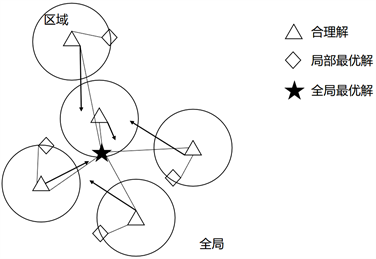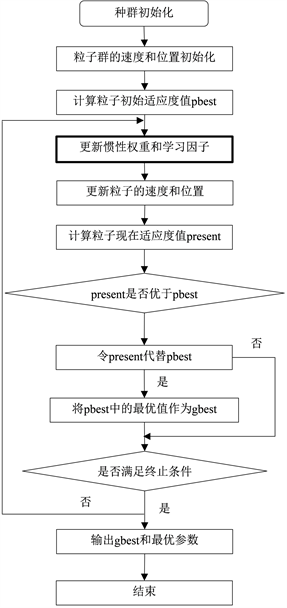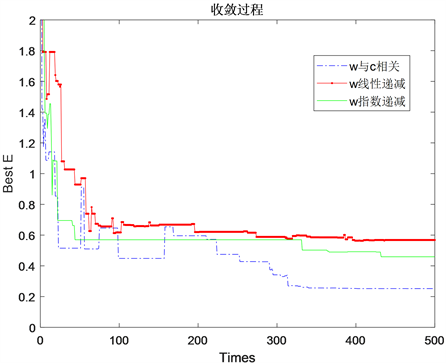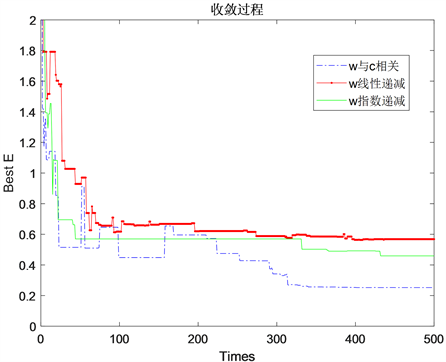﻿ 改进实现主动吸收的IIR数字滤波器优化算法

# 改进实现主动吸收的IIR数字滤波器优化算法 Improved IIR Digital Filter Optimization Algorithm for Active Absorption

Abstract: In the ocean engineering test basin, during the model test, the wave generated by the wave-makers generates reflected waves after being reflected from the basin wall and structural model, which propagates to the wave-making plates and reflects again to generate the secondary reflected wave. The secondary reflected wave interferes with the wave field of the basin, thus reducing the accuracy of the test, which is unfavorable to the test. An active absorption system is often used to eliminate the secondary reflection wave. The force-feedback type active absorption system takes the ratio of the force to the stroke of the wave-making paddles as the absorption transfer function, and controls the input and output of the system in real time, so that the generated wave gradually approaches the ideal wave. In order to maintain the optimal balance between the stroke and force of the wave-making paddles, IIR digital filter based on the theoretical transfer function is designed to realize the active absorption control. To design IIR digital filter, particle swarm optimization algorithm is a very efficient design method, the algorithm is simple and has fast calculation speed, but the algorithm in the optimization process could be limited to the local optimum, and can’t get the global optimal solution. In this paper, a new kind of method is used to update the particle velocity based on inertia weight to improve the learning factor. Inertia weight and learning factor have a nonlinear function relation. Compared with the improved method of linear decrease and nonlinear decrease of inertia weight, the simulation results show that this method has fast convergence speed, fewer iteration times, higher solving efficiency and optimal optimization results.

1. 引言

IIR数字滤波器的设计就是使得所求的幅频特性尽可能地逼近理想幅频特性，设计方法主要分为两类：一是通过变换方法将模拟滤波器转换为数字滤波器，但是该方法设计效果不理想；二是直接利用优化算法设计数字滤波器，在优化准则下求解最优的滤波器系数，使设计的滤波器性能最优。目前，利用优化算法优化设计IIR数字滤波器是国内外研究的重点。

2. 吸收传递函数与数字滤波器

${c}_{0}=\frac{A}{X}$. (1)

${c}_{f}=\frac{F}{X}$. (2)

${c}_{f}={c}_{0}\frac{1}{i\omega }\frac{1}{{Z}_{d}+{Z}_{f}}$. (3)

3. 粒子群优化算法

IIR数字滤波器在信号处理领域有非常重要的作用，目前滤波器设计方法主要分为两种：一是先设计模拟滤波器，然后利用脉冲响应不变法、阶跃响应不变法、双线性变换法等将其转换为数字滤波器，这种方法比较简单，但是设计误差大，结果不理想；二是直接使用优化算法，优化算法可以使得设计的滤波器性能最优，比如最小二乘法、粒子群算法、量子粒子群算法、人工蜂群算法、遗传算法、量子遗传算法等，其中，粒子群优化算法PSO (Partical Swarm Optimization)研究了鸟类等粒子所表现出的群体行为，如图1，将待优化的目标变量比作鸟类等粒子的位置信息，将目标函数的解比作鸟群要找的食物，最终通过鸟群内部一系列操作得到问题的最优解，该算法能够将更多的粒子聚集在最优粒子附近，从而增强优化能力。粒子群优化算法在解决非线性问题方面具有很好的表现，而且算法设计简单可行，成为解决许多非线性优化问题的有力工具。Figure 1. Principle of particle swarm optimization

$V=w\ast V+c1\ast \text{rand}\left(\text{pBest}-\text{Present}\right)+c2\ast \text{rand}\left(\text{gBest}-\text{Present}\right)$,(4)

$\text{Present}=\text{Present}+V$.(5)

IIR滤波器的传递函数可以表示为 ：

$H\left(z\right)={A}_{0}\underset{k=1}{\overset{N}{\prod }}\frac{1+{a}_{k}{z}^{-1}+{b}_{k}{z}^{-2}}{1+{c}_{k}{z}^{-1}+{d}_{k}{z}^{-2}}$. (6)

$H\left({\text{e}}^{j\omega }\right)={A}_{0}\underset{k=1}{\overset{N}{\prod }}\frac{1+{a}_{k}{z}^{-j\omega }+{b}_{k}{z}^{-2j\omega }}{1+{c}_{k}{z}^{-j\omega }+{d}_{k}{z}^{-2j\omega }}={A}_{0}G\left({\text{e}}^{j\omega }\right)$.(7)

$E=\underset{i=1}{\overset{M}{\sum }}{\left[|H\left({\text{e}}^{j\omega }\right)|-|{H}_{d}\left({\text{e}}^{j\omega }\right)|\right]}^{2}$ 为最小。从该式可以看出，目标函数E是一种非线性函数，它与增益A0

E可以表示为A0akbkckdk的函数，即：

$E=\underset{i=1}{\overset{M}{\sum }}{\left[|{A}_{0}|\cdot |G\left({\text{e}}^{j\omega }\right)|-|{H}_{d}\left({\text{e}}^{j\omega }\right)|\right]}^{2}$. (8)

${A}_{0}=\underset{i=1}{\overset{M}{\sum }}|G\left({\text{e}}^{j\omega }\right)|\cdot |{H}_{d}\left({\text{e}}^{j\omega }\right)|/\underset{i=1}{\overset{M}{\sum }}{|G\left({\text{e}}^{j\omega }\right)|}^{2}$. (9)

$F=E=\underset{i=1}{\overset{M}{\sum }}{\left[|H\left({\text{e}}^{j\omega }\right)|-|{H}_{d}\left({\text{e}}^{j\omega }\right)|\right]}^{2}$. (10)

4. 改进惯性权重和学习因子

1) 线性递减权重法

$w={w}_{\mathrm{max}}-\left({w}_{\mathrm{max}}-{w}_{\mathrm{min}}\right)\cdot \left(t/T\right)$. (11)

2) 非线性递减权重法

$w={w}_{\mathrm{min}}+\left({w}_{\mathrm{max}}-{w}_{\mathrm{min}}\right)\cdot \mathrm{exp}\left(-20{\left(t/T\right)}^{6}\right)$. (12)

3) 基于惯性权重改进学习因子

$c1=0.5{w}^{2}+w+0.5$, (13)

$c2=2.5-c1$. (14)

5. 实验仿真与分析Figure 2. Flow chart of particle swarm optimization

$H\left({\text{e}}^{j\omega }\right)=\left\{\begin{array}{cc}\begin{array}{l}1\\ 0.5\\ 0\end{array}& \begin{array}{l}0\le \omega \le 0.4\pi \\ \omega =0.45\pi \\ 0.5\pi \le \omega \le \pi \end{array}\end{array}$. (15)

${H}_{d}\left({\text{e}}^{j\omega }\right)=\left\{\begin{array}{cc}\begin{array}{l}0\\ 1\\ 0.5\end{array}& \begin{array}{l}0\le \omega \le 0.28\pi ,\text{\hspace{0.17em}}\text{\hspace{0.17em}}0.72\pi \le \omega \le \pi \\ 0.32\pi \le \omega \le 0.68\pi \\ \omega =0.30\pi ,\text{\hspace{0.17em}}\text{\hspace{0.17em}}\omega =0.70\pi \end{array}\end{array}$. (16)Figure 3. Flow chart of particle swarm optimizationTable 1. Lowpass IIR filter simulation design resultsTable 2. Bandpass IIR digital filter simulation design resultsFigure 4. The iterative process of the three improvement methods for lowpass IIR digital filterFigure 5. The iterative process of the three improvement methods for bandpass IIR digital filter

6. 结论

 兰波, 周德才, 胡定健. 波浪水池圆弧斜坡式消波装置改型试验研究[C]//《水动力学研究与进展》编委会, 中国造船工程学会, 江苏大学. 第二十九届全国水动力学研讨会论文集(上册). 上海: 上海《水动力学研究与进展》杂志社, 2018: 370-376.

 Spinneken, J. and Swan, C. (2009) Wave Generation and Absorption Using Force-Controlled Wave Machines. Proceedings of the 19th International Offshore and Polar Engineering Conference, 3, 397-404.

 Teng, B. and Cong, P.W. (2017) A Novel Decomposition of the Quadratic Transfer Function (QTF) for the Time-Domain Simulation of Non-Linear Wave Forces on Floating Bodies. Applied Ocean Research, 65, 112-128.
https://doi.org/10.1016/j.apor.2017.03.016

 Spinneken, J. (2010) Wave Generation and Absorption Using Force-Feedback Control. Thesis, Imperial College London, London.

 O’Boyle, L. and Whittaker, T. (2017) Methods to Enhance the Performance of a 3D Coastal Wave Basin. Ocean Engineering, 135, 158-169.
https://doi.org/10.1016/j.oceaneng.2017.03.006

 陆慧慧. 基于智能优化算法的数字滤波器设计[D]: [硕士学位论文]. 哈尔滨: 哈尔滨工程大学, 2016.

 Spinneken, J. and Swan, C. (2010) Theoretical Transfer Function of Force-Controlled Wave Machines. International Offshore and Polar Engineering Conference, Beijing, China, June 2010.

 曹晓月, 张旭秀. 学习因子随权重调整的混合粒子群算法[J]. 计算机技术与发展, 2020, 283(11): 36-42.

 赵远东, 方正华. 带有权重函数学习因子的粒子群算法[J]. 计算机应用, 2013, 33(8): 2265-2268.

Top